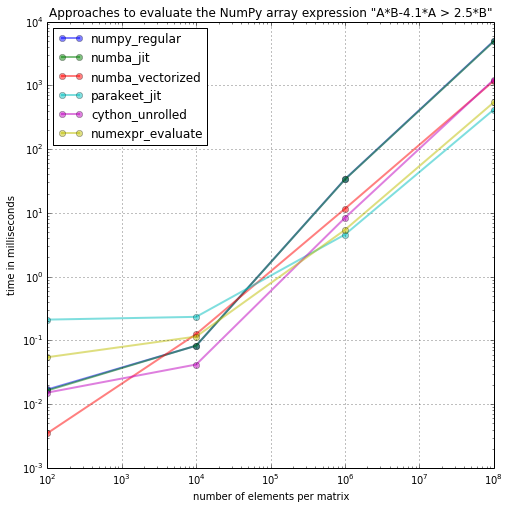# Day 7.2 - One Python Benchmark per Day¶

## Using just-in-time compilers for speeding up NumPy array expressions¶

This is a follow-up to day 7, where I compared NumPy with Numexpr for various different operators. Now, I only want focus on one expression that was listed in the Numexpr documentation (which supposedly show off its strengths), and compare the performance of Numexpr to the two other JIT (just-in-time) compilers Numba and parakeet.

For this benchmark, we will compare different arithmetic operators for simple matrix calculations using NumPy arrays to their Numexpr equivalents.

### Test function in NumPy¶

In :
import numpy as np

In :
def numpy_regular(A, B):
return(A*B-4.1*A > 2.5*B)


### Test function in Numexpr¶

Numexpr is a "fast numerical expression evaluator for NumPy". It has a internal Virtual Machine to rewrite code more efficiently by breaking down operations into smaller chunks for the CPU cache. By using a JIT (just-in-time compiler), compilation at runtime is not necessary.

The usage is quite simple, we just have to provide the numerical expression that we want to evaluate as a string to the evaluate function, e.g.,

import numexpr
import numpy
a = numpy.array([[1, 2]])
numexpr.evaluate('a ** 2')
>> array([[1, 4]], dtype=int64)
In :
import numexpr as ne

In :
def numexpr_evaluate(A, B):
return ne.evaluate('A*B-4.1*A > 2.5*B')


### Test function in Numba¶

Numba is using the LLVM compiler infrastructure for compiling Python code to machine code. Its strength is to work with NumPy arrays to speed-up the code. If you want to read more about Numba, please see refer to the original website and documentation.

Continuum Analytics write on their website that "the NumPy array ufunc$^1$ support in nopython node is incomplete at this time. Most if not all ufuncs should work in object mode though."

$^1$ "A universal function (or ufunc for short) is a function that operates on ndarrays in an element-by-element fashion
(http://docs.scipy.org/doc/numpy/reference/ufuncs.html)

In :
from numba import jit

In :
@jit
def numba_jit(A, B):
return A*B-4.1*A > 2.5*B

In :
from numba import vectorize

In :
@vectorize(['u1(float64, float64)'])
def numba_vectorized(A,B):
return A*B+4.1*A > 2.5*B


### Test function in parakeet¶

Similar to Numba, parakeet is a Python compiler that optimizes the runtime of numerical computations based on the NumPy data types, such as NumPy arrays.

The usage is also similar to Numba where we just have to put the jit decorator on top of the function we want to optimize.

In :
from parakeet import jit as para_jit

In :
@para_jit
def parakeet_jit(A, B):
return A*B-4.1*A > 2.5*B


### Test function in Cython¶

Maybe we can speed things up a little bit via Cython's C-extensions for Python. Cython is basically a hybrid between C and Python and can be pictured as compiled Python code with type declarations.
Since we are working in an IPython notebook here, we can make use of the very convenient IPython magic: It will take care of the conversion to C code, the compilation, and eventually the loading of the function.

Note that the static type declarations that we add via cdef are note required for Cython to work, but it will speed things up tremendously.

In :
%load_ext cythonmagic

In :
%%cython
import numpy as np
cimport numpy as np
cimport cython
@cython.boundscheck(False)
@cython.wraparound(False)
cpdef cython_unrolled(double[:, :] A, double[:, :] B):
cdef long i, j, rows, cols
cdef double[:, :] C
rows = A.shape
cols = A.shape
C = np.empty((rows,cols,))
for i in xrange(rows):
for j in xrange(cols):
C[i,j] = A[i,j]*B[i,j] + 4.1*A[i,j] > 2.5*B[i,j]

return np.asarray(C)


# Timing¶

In :
import timeit

orders_n = [10**n for n in range(1, 5)]

funcs = ['numpy_regular', 'numba_jit', 'numba_vectorized',
'parakeet_jit', 'cython_unrolled', 'numexpr_evaluate'
]

timings = {f:[] for f in funcs}

for n in orders_n:
for f in funcs:
A = np.random.rand(n,n)
B = np.random.rand(n,n)
timings[f].append(min(timeit.Timer('%s(A, B)' %f,
'from __main__ import A, B, %s' %f)
.repeat(repeat=3, number=10)))


## Setting up plots¶

In :
import platform
from llvm import __version__ as llvm__version__
from parakeet import __version__ as parakeet__version__
from numba import __version__ as numba__version__

def print_sysinfo():
print '\nsystem   :', platform.system()
print 'release  :', platform.release()
print 'machine  :', platform.machine()
print 'processor:', platform.processor()
print 'interpreter:', platform.architecture()

print '\nPython version', platform.python_version()
print 'compiler', platform.python_compiler()
print 'NumPy version', np.__version__
print 'Numexpr version', ne.__version__
print 'parakeet version', parakeet__version__
print 'Numba version', numba__version__
print 'llvm version', llvm__version__
print '\n\n'

In :
import prettytable

def summary_table(funcs):
fit_table = prettytable.PrettyTable(['n=%s' %orders_n[-1],
'function' ,
'rel. performance gain'])
fit_table.align['function'] = 'l'
for f in funcs:
(timings['numpy_regular'][-1]/timings[f][-1]))])
print(fit_table)

In :
%matplotlib inline

In :
import matplotlib.pyplot as plt

def plot_figures(funcs):
fig = plt.figure(figsize=(8,8))
for f in funcs:
plt.plot([i**2 for i in orders_n], [i*100 for i in timings[f]],
alpha=0.5, label='%s' %f, marker='o', lw=2)
plt.legend(loc='upper left')
plt.xscale('log')
plt.yscale('log')
plt.grid()
plt.xlabel('number of elements per matrix')
plt.ylabel('time in milliseconds')
plt.title('Approaches to evaluate the NumPy array expression "A*B-4.1*A > 2.5*B"')

plt.show()


# Results¶

In :
print_sysinfo()
summary_table(funcs)
plot_figures(funcs)

system   : Darwin
release  : 13.1.0
machine  : x86_64
processor: i386
interpreter: 64bit

Python version 2.7.6
compiler GCC 4.0.1 (Apple Inc. build 5493)
NumPy version 1.8.1
Numexpr version 2.4
parakeet version 0.24
Numba version 0.13.1
llvm version 0.12.4

+---------+------------------+-----------------------+
| n=10000 | function         | rel. performance gain |
+---------+------------------+-----------------------+
|         | numpy_regular    |         1.00x         |
|         | numba_jit        |         1.02x         |
|         | numba_vectorized |         4.23x         |
|         | parakeet_jit     |         11.92x        |
|         | cython_unrolled  |         4.04x         |
|         | numexpr_evaluate |         9.17x         |
+---------+------------------+-----------------------+In :
import multiprocessing
print('This benchmark was done on a machine with %s CPUs', multiprocessing.cpu_count())

This benchmark was done on a machine with 2 CPUs

In [ ]: